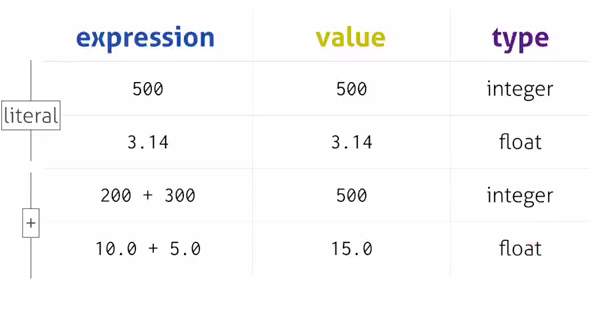# 2.10. Statements and Expressions¶

A statement is an instruction that the Python interpreter can execute. You have only seen the assignment statement so far. Some other kinds of statements that you’ll see in future chapters are while statements, for statements, if statements, and import statements. (There are other kinds too!)

An expression is a combination of literals, variable names, operators, and calls to functions. Expressions need to be evaluated. The result of evaluating an expression is a value or object.If you ask Python to print an expression, the interpreter evaluates the expression and displays the result.

In this example len is a built-in Python function that returns the number of characters in a string.

The evaluation of an expression produces a value, which is why expressions can appear on the right hand side of assignment statements. A literal all by itself is a simple expression, and so is a variable.

In a program, anywhere that a literal value (a string or a number) is acceptable, a more complicated expression is also acceptable. Here are all the kinds of expressions we’ve seen so far:

literal

e.g., “Hello” or 3.14

variable name

e.g., x or len

operator expression

<expression> operator-name <expression>

function call expressions

<expression>(<expressions separated by commas>)

Notice that operator expressions (like + and *) have sub-expressions before and after the operator. Each of these can themselves be simple or complex expressions. In that way, you can build up to having pretty complicated expressions.

Similarly, when calling a function, instead of putting a literal inside the parentheses, a complex expression can be placed inside the parentheses. (Again, we provide some hidden code that defines the functions square and sub).

With a function call, it’s even possible to have a complex expression before the left parenthesis, as long as that expression evaluates to a function object. For now, though, we will just use variable names (like square, sub, and len) that are directly bound to function objects.

It is important to start learning to read code that contains complex expressions. The Python interpreter examines any line of code and parses it into components. For example, if it sees an = symbol, it will try to treat the whole line as an assignment statement. It will expect to see a valid variable name to the left of the =, and will parse everything to the right of the = as an expression. It will try to figure out whether the right side is a literal, a variable name, an operator expression, or a function call expression. If it’s an operator expression, it will further try to parse the sub-expressions before and after the operator. And so on. You should learn to parse lines of code in the same way.

In order to evaluate an operator expression, the Python interpreter first completely evaluates the expression before the operator, then the one after, then combines the two resulting values using the operator. In order to evaluate a function call expression, the interpreter evaluates the expression before the parentheses (i.e., it looks up the name of the function). Then it tries to evaluate each of the expressions inside the parentheses. There may be more than one, separated by commas. The values of those expressions are passed as inputs to the function when the function is called.

If a function call expression is a sub-expression of some more complicated expression, as square(x) is in sub(square(y), square(x)), then the return value from square(x) is passed as an input to the sub function. This is one of the tricky things that you will have to get used to working out when you read (or write) code. In this example, the square function is called (twice) before the sub function is called, even though the sub function comes first when reading the code from left to right. In the following example we will use the notation of -add- to indicate that Python has looked up the name add and determined that it is a function object.

x = 5
y = 7